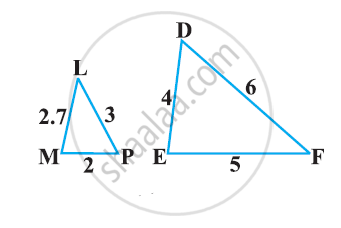# State which pair of triangles in the following figure are similar? Write the similarity criterion used by you for answering the question and also write the pairs of similar triangles in the symbolic form - Mathematics

State which pair of triangles in the following figure are similar? Write the similarity criterion used by you for answering the question and also write the pairs of similar triangles in the symbolic form:#### Solution 1

The given triangles are not similar as the corresponding sides are not proportional.

#### Solution 2

In ΔLMP and ΔDEF, we have
LM = 2.7, MP = 2, LP = 3, EF = 5, DE = 4, DF = 6
MP/DE = 2/4 = 1/2
PL/DF = 3/6 = 1/2
LM/EF= 2.7/5 = 27/50
Here, MP/DE = PL/DF ≠ LM/EF
Hence, ΔLMP and ΔDEF are not similar.

Concept: Criteria for Similarity of Triangles
Is there an error in this question or solution?

#### APPEARS IN

NCERT Class 10 Maths
Chapter 6 Triangles
Exercise 6.3 | Q 1.3 | Page 139